# 程序员最近都爱上了这个网站  程序员们快来瞅瞅吧！  it98k网:it98k.com出租广告位,需要合作请联系站长

+关注

2021-10(17)

2021-11(17)

## 【Python数据科学快速入门系列 | 06】Matplotlib数据可视化基础入门(一)# 《Python数据科学快速入门系列》快速导航：

• 博客简介：专注AIoT领域，追逐未来时代的脉搏，记录路途中的技术成长！
• 博主社区：AIoT机器智能, 欢迎加入！
• 专栏简介：从0到1掌握数据科学常用库Numpy、Matploblib、Pandas。
• 面向人群：AI初级学习者

# 1. Matplotlib简介

Matplotlib是一个数据可视化综合绘图库，python三剑客(Numpy、Matplotlib、Pandas)之一，用于创建静态图、动态图和Python中的交互式可视化图像。

# 2. Matplotlib的安装

``````pip install matplotlib
``````

``````conda install matplotlib
``````

# 3. Matplotlib的基础使用

## 3.1 第一个Matplot例子：绘制折线图

``````#引入matplotlib.pyplot绘图库
from matplotlib import pyplot as plt

#创建一个只有单个子图的画布
fig,ax=plt.subplots()#Create a figure containing a single axes.

#4个坐标点的坐标分别是(1,1),(2,4),(3,2),(4,3)
ax.plot([1,2,3,4],[1,4,2,3]);#Plot some data on the axes.

plt.show()
``````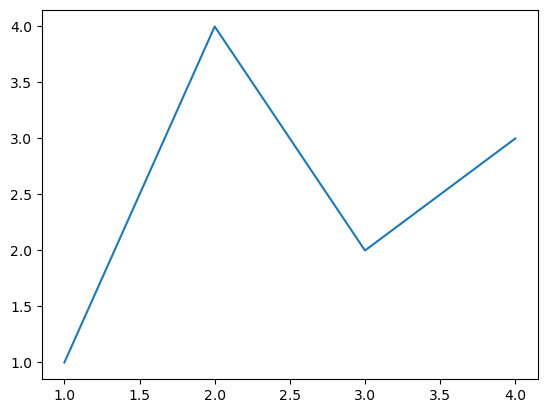• 首先创建了一个画布fig
• 然后创建了一个绘图区域ax(axes)，这个绘图区域有2个坐标轴axis，分别在横轴和纵轴。
• 然后绘图区域对象ax调用了方法plot绘制了4个坐标点，形成折线图。

## 3.2 编码风格

``````#引入matplotlib.pyplot绘图库
from matplotlib import pyplot as plt

#4个坐标点的坐标分别是(1,1),(2,4),(3,2),(4,3)
plt.plot([1,2,3,4],[1,4,2,3]);#Plot some data on the axes. b

plt.show()
``````• 隐式创建一个画布，并创建一个绘图区域
• 然后绘制4个坐标点，绘制折线图

## 3.2 绘图参数详解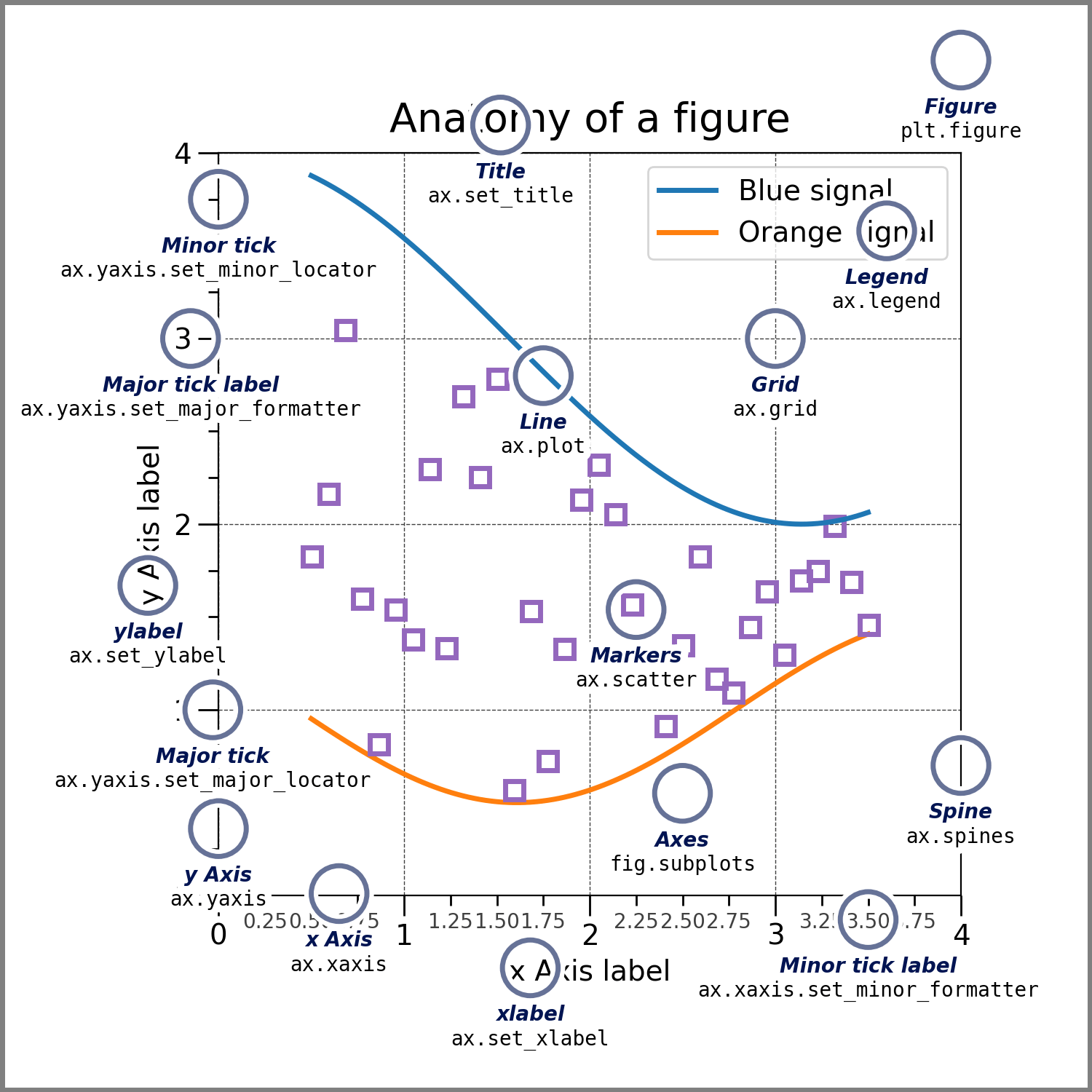### 3.2.1 Figure画布

``````from matplotlib import pyplot as plt

#创建空白画布
fig = plt.figure()#an empty figure with no Axes
plt.show()
``````
``````<Figure size 640x480 with 0 Axes>
``````
``````from matplotlib import pyplot as plt

#创建单个绘图区域的画布
fig,ax=plt.subplots()#a figure with a single Axes
plt.show()
``````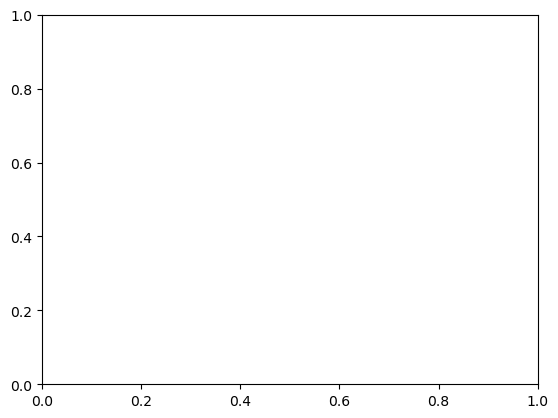``````from matplotlib import pyplot as plt

#创建2x2四个绘图区域的画布
fig,axs=plt.subplots(2,2)#a figure with a 2x2 grid of Axes
plt.show()
``````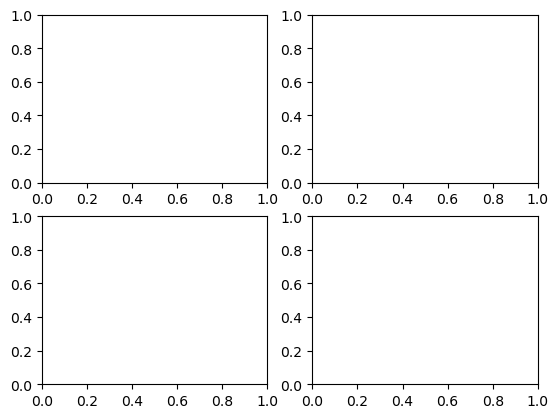### 3.2.2 Axes绘图区域与Axis坐标轴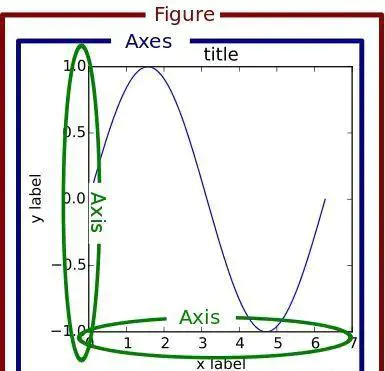### 3.2.3 输入数据的类型

x, y array-like or scalar

### 3.2.4 绘图样式

#### 3.2.4.1 标准表示

• color

支持颜色英文名称和十六进制颜色代码，例如black和#000000

• linewidth

浮点类型

• linestyle

linestyledescription
`'-'` or `'solid'`实线
`'--'` or `'dashed'`杠虚线
`'-.'` or `'dashdot'`点杠虚线
`':'` or `'dotted'`点虚线
`'none'`, `'None'`, `' '`, or `''`
• marker

marker样式非常多，更多样式参考官方文档:https://matplotlib.org/stable/api/markers_api.html#module-matplotlib.markers

markersymboldescription
`"."`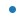point
`","`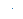pixel
`"o"`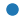circle
`"v"`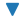triangle_down
`"^"`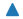triangle_up
`"<"`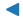triangle_left
`">"`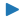triangle_right
`"1"`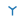tri_down
`"2"`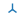tri_up
`"3"`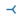tri_left
`"4"`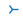tri_right
`"8"`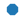octagon
`"s"`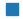square
`"p"`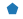pentagon
`"P"`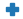plus (filled)
`"*"`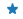star
`"h"`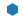hexagon1
`"H"`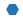hexagon2
`"+"`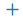plus
`"x"`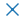x
`"X"`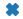x (filled)
`"D"`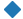diamond
`"d"`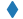thin_diamond
`"|"`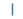vline
`"_"`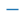hline
``````"""

"""

import matplotlib as mpl
import matplotlib.pyplot as plt
import numpy as np

fig, ax = plt.subplots(figsize=(8, 5))
# 生成0~2π之间的等差数列，数据元素为30个
x = np.linspace(0, 2*np.pi, 30)
y1 = np.cos(x)
y2 = np.sin(x)
y3 = np.cos(2*x)
y4 = np.sin(2*x)
# 绘制余弦曲线，颜色为黑色，线宽3，线样式为杠虚线，点标记为小三角
ax.plot(x, y1, color='#000000', linewidth=3, linestyle='--', marker='^')
# 绘制正弦函数曲线，颜色为橙色，线宽2，点标记为星号
l, = ax.plot(x, y2, color='orange', linewidth=2, marker='*')
# 单独设置线样式为点虚线
l.set_linestyle(':')
# 绘制2x余弦函数曲线，线宽为8，线样式为杠点虚线
ax.plot(x, y3, color='red', linewidth=6, linestyle='-.')
# 绘制2x正弦函数曲线，颜色为绿色，线宽为1，线样式为实线
ax.plot(x, y4, color='green', linewidth=2, linestyle='-')
plt.show()

``````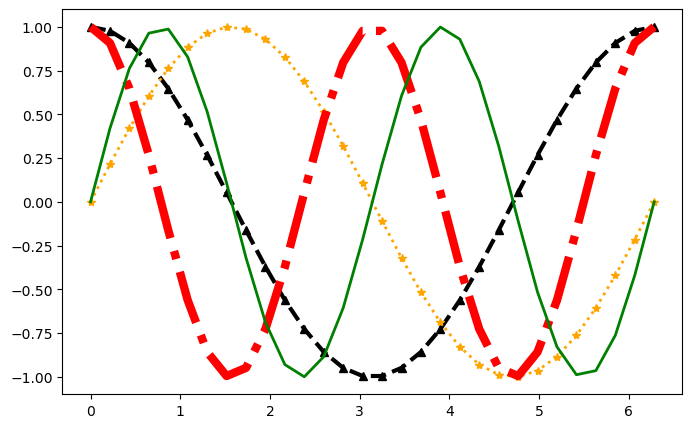#### 3.2.4.2 简写表示

``````plot([x], y, [fmt], *, data=None, **kwargs)
``````
``````fmt = '[marker][line][color]'

fmt = '[color][marker][line]'
``````

fmt的内容本身没有限定顺序，可以自由组合。

line指的是line_style，marker和line_style的取值和上面的表描述是一样的，颜色代码简写表示如下：

`'b'`蓝色的
`'g'`绿色
`'r'`红色的
`'c'`青色
`'m'`品红
`'y'`黄色
`'k'`黑色的
`'w'`白色的
``````import matplotlib as mpl
import matplotlib.pyplot as plt
import numpy as np

# 生成0~2π之间的等差数列，数据元素为30个
x = np.linspace(0, 2*np.pi, 30)
y1 = np.cos(x)
y2 = np.sin(x)
y3 = np.cos(2*x)

# g-green,o-cycle圆点标记，--为杠杠虚线，其它样式需要单独指定
plt.plot(x, y1, 'go--', linewidth=2, markersize=6)
# c-青色，^-三角标志，:为点虚线
plt.plot(x, y2, 'c^:', linewidth=2, markersize=3)
# b-blue，*-star标志，-.为杠点虚线
plt.plot(x, y3, 'b*-.', linewidth=2, markersize=3)
``````
``````[<matplotlib.lines.Line2D at 0x7fd1e088b6a0>]
``````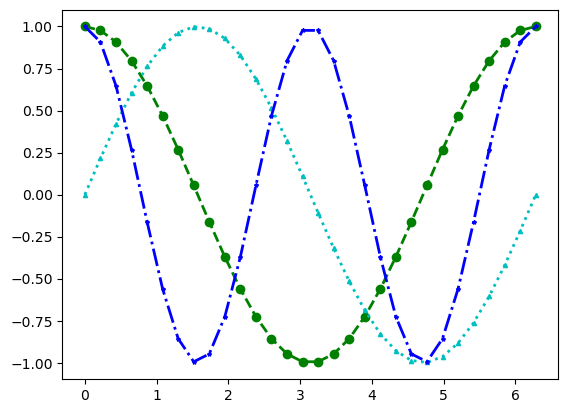【Python数据科学快速入门系列 | 06】Matplotlib数据可视化基础入门(二)

— 博主热门专栏推荐 —30 0

pdf(new) 更多>